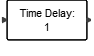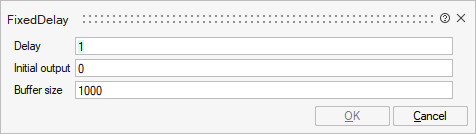# FixedDelay

This block delays the input signal by a specified amount of time.## Library

Activate/Dynamical

## Description

The Fixed Delay block delays an input by a specified amount of time. You can use the block to simulate a time delay in a continuous system.

At the start of a simulation, the block outputs the "Initial Output" parameter until the simulation time exceeds the Delay parameter, when the block begins generating the delayed input.

The Delay parameter must be non-negative.

When the desired delayed time doesn't correspond to any step of the input signal, the block performs a linear interpolation based on the stored signal in the buffer. E.g. current time is 5, fixed step size is 1, parameter Delay is 1.5, so interpolation happens based on inputs at time 3 and 4. When the parameter Delay is less than current step size, the output is the last input. E.g. current time is 5, fixed step size is 1, parameter Delay is 0.5, the output is the intput at time 4.

## ParametersNameLabelDescriptionData TypeValid Values

T

Delay

Real value. The amount of simulation time that the input signal is delayed before being propagated to the output. The value must be non-negative.

Matrix

init

Initial output

The output generated by the block between the start of the simulation and the Time Delay.

Initial output value can be a cell composed of a time vector and an initial value matrix.

Here is an example of a vector initial output value: {[-3,-2,-1],[4,5,1]} where the first element of the cell is the time vector and the second element is the initial output matrix.

If the initial output data is a matrix, output data matrices at different time instants concatenated.

For example in {[-3,-2,-1],[4,5,1,3,7,6]} the value of output when the delay is 3 second is [4,5].

N

Buffer size

Integer value. The initial memory allocation for the number of points to store.

Scalar

## Ports

NameTypeDescriptionIO TypeNumber

Port 1

explicit

input

1

Port 2

explicit

output

1

NameValueDescription

always active

yes

direct-feedthrough

no

zero-crossing

no

mode

no

continuous-time state

no

discrete-time state

no